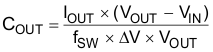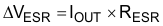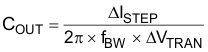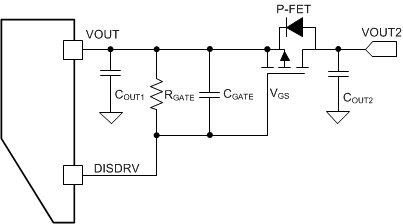SLVSDA7E February   2017  – August 2019

PRODUCTION DATA.

1. Features
2. Applications
3. Description
1.     Device Images
4. Revision History
5. Device Comparison Table
6. Pin Configuration and Functions
7. Specifications
8. Detailed Description
1. 8.1 Overview
2. 8.2 Functional Block Diagram
3. 8.3 Feature Description
4. 8.4 Device Functional Modes
9. Application and Implementation
1. 9.1 Application Information
2. 9.2 Typical Application
1. 9.2.1 Design Requirements
2. 9.2.2 Detailed Design Procedure
3. 9.2.3 Setting the Current Limit
4. 9.2.4 Setting the Output Voltage
5. 9.2.5 TPS61178 Application Waveform
3. 9.3 System Examples
10. 10Power Supply Recommendations
11. 11Layout
12. 12Device and Documentation Support
1. 12.1 Device Support
2. 12.2 Documentation Support
5. 12.5 Community Resources
7. 12.7 Electrostatic Discharge Caution
8. 12.8 Glossary
13. 13Mechanical, Packaging, and Orderable Information

• RNW|13

#### 9.2.4.2 Selecting the Output Capacitors

The output capacitor is mainly selected to meet the requirements at load transient or steady state. Then the loop is compensated for the output capacitor selected. The output ripple voltage is related to the equivalent series resistance (ESR) of the capacitor and its capacitance. Assuming a capacitor with zero ESR, the minimum capacitance needed for a given ripple can be calculated by Equation 12:

Equation 12.where

• COUT is the output capacitor
• IOUT is the output current
• VOUT is the output voltage
• VIN is the input voltage
• ΔV is the output voltage ripple required
• ƒSW is the switching frequency

The additional output ripple component caused by ESR is calculated by Equation 13:

Equation 13.where

• ΔVESR is the output voltage ripple caused by ESR
• RESR is the resistor in series with the output capacitor

For the ceramic capacitor, the ESR ripple can be neglected. However, for the tantalum or electrolytic capacitors, it must be considered if used.

The minimum ceramic output capacitance needed to meet a load transient requirement can be estimated using Equation 14:

Equation 14.where

• ΔISTEP is the transient load current step
• ΔVTRAN is the allowed voltage dip for the load current step
• ƒBW is the control loop bandwidth (i.e., the frequency where the control loop gain crosses zero)

Care must be taken when evaluating a ceramic capacitor’s derating under the DC bias. Ceramic capacitors can derate by as much as 70% of its capacitance at its rated voltage. Therefore, enough margins on the voltage rating should be considered to ensure adequate capacitance at the required output voltage.

In applications of TPS61178x, it is recommended to run the converter with a reasonable amount of effective output capacitance, for instance 3 x 22-μF X5R or X7R MLCC capacitors connected in parallel.

If the load disconnect FET is connected, the output capacitor should be split shown in Figure 23. COUT2 should be no larger than 10 x COUT1 to avoid the inrush current when turning on the disconnect FET.Figure 23. Output Capacitor Configuration with the Load Disconnect FET